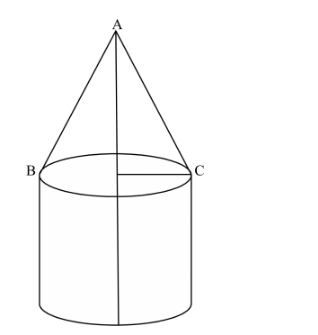# A tent of height 77 dm is in the form a right circular cylinder of diameter 36 m and height 44 dmQuestion:

A tent of height $77 \mathrm{dm}$ is in the form a right circular cylinder of diameter $36 \mathrm{~m}$ and height $44 \mathrm{dm}$ surmounted by a right circular cone. Find the cost of the canvas at Rs $3.50$ per $\mathrm{m}^{2}$. [Use $\pi=22 / 7$ ]

Solution:

The height of the tent is 77dm = 7.7m. The height of the upper portion of the tent is

$44 \mathrm{dm}=4.4 \mathrm{~m}$. Therefore, the height of the cylindrical part is $77-44=33 \mathrm{dm}=3.3 \mathrm{~m}$. The radius of the cylindrical part is $\frac{36}{2}=18 \mathrm{~m}$.Let the slant height of the cone part is l m. Then, we have

$l^{2}=(18)^{2}+(3.3)^{2}$

$\Rightarrow l^{2}=324+10.89=334.89$

$\Rightarrow \quad l=18.3$

Therefore, the slant height of the cone part is 18.3 m.

The curved surface area of the cylindrical part is

$S=2 \pi \times 18 \times 4.4 \mathrm{~m}^{2}$

The curved surface area of the cone part is

$S_{1}=\pi \times 18 \times 18.3 \mathrm{~m}^{2}$

Therefore, the total curved surface area of the tent is

$S+S_{1}=2 \pi \times 18 \times 4.4+\pi \times 18 \times 18.3$

$=18 \pi(8.8+18.3)$

$=18 \pi \times 27.10$

The cost of canvas per $\mathrm{m}^{2}$ is Rs $3.50$. Hence, the total cost for canvas in Rs is

$=18 \times \frac{22}{7} \times 27.10 \times 3.50$

$=5365.80$

Hence total cost is Rs.5365.80